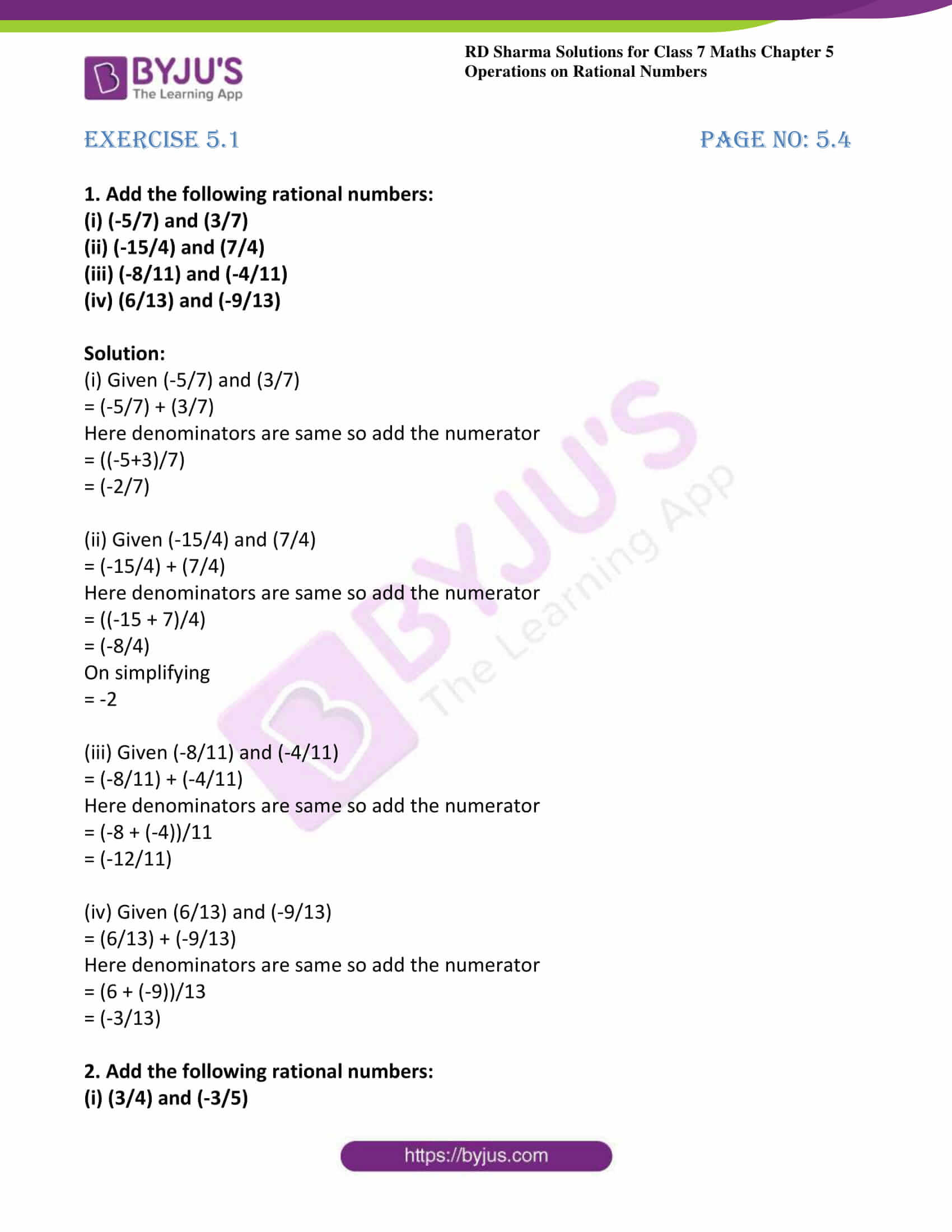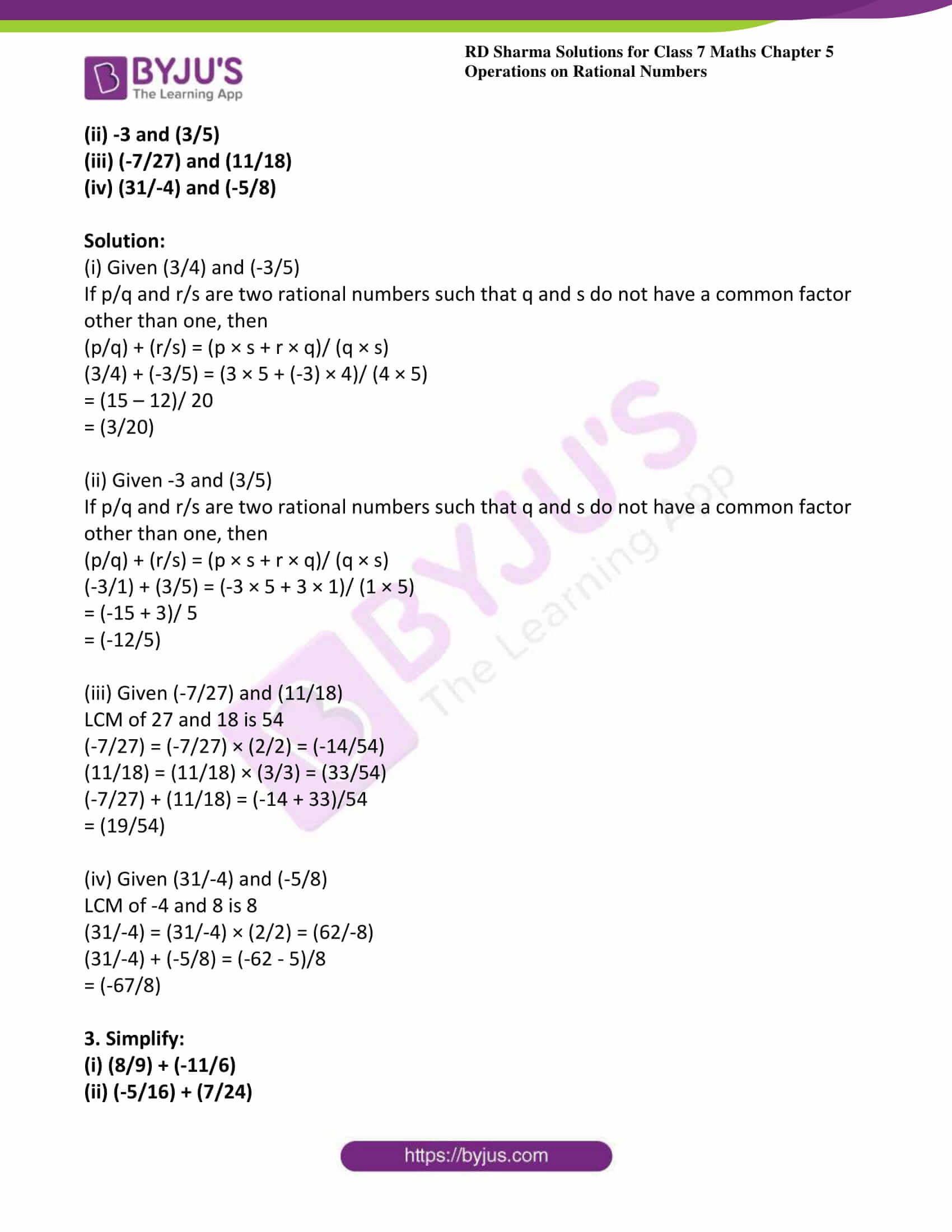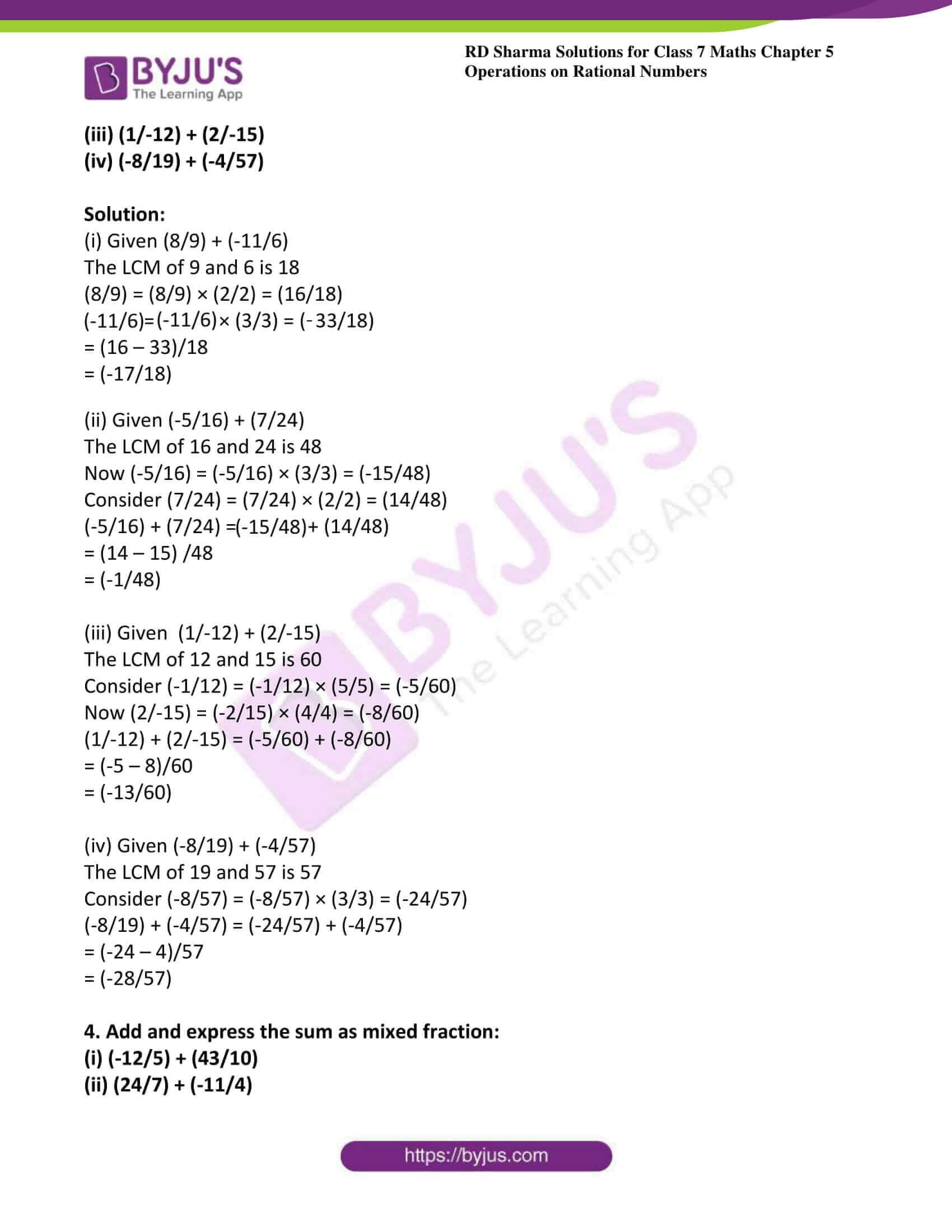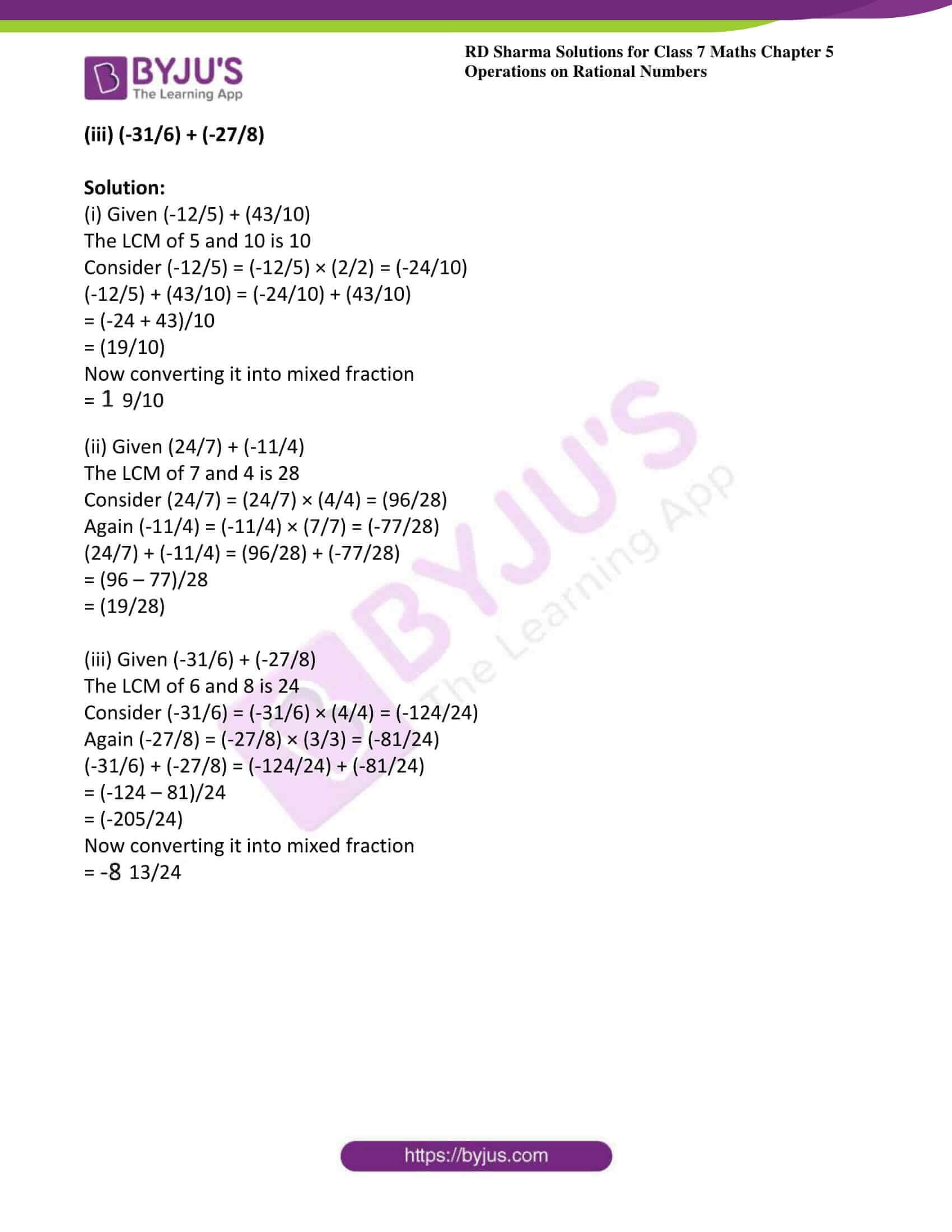# RD Sharma Solutions For Class 7 Maths Exercise 5.1 Chapter 5 Operations On Rational Numbers

The main objective of providing exercise-wise solutions in PDF is to help students speed up their exam preparation. The solutions are designed based on the latest CBSE syllabus and exam pattern. Students can refer and download the RD Sharma Solutions for Class 7 Chapter 5 Operations on Rational Numbers Exercise 5.1 from the link provided here. Our specialist tutors formulate these exercises to help you with your exam preparation to achieve good marks in Maths. This exercise contains four questions with many sub-questions and all are based on basic concepts related to operations on rational numbers. By practising RD Sharma Solutions for class 7, students will be able to grasp the concepts perfectly.

## Download the PDF of RD Sharma Solutions For Class 7 Maths Chapter 5 – Operations On Rational Numbers Exercise 5.1### Access answers to Maths RD Sharma Solutions For Class 7 Chapter 5 – Operations On Rational Numbers Exercise 5.1

1. Add the following rational numbers:

(i) (-5/7) and (3/7)

(ii) (-15/4) and (7/4)

(iii) (-8/11) and (-4/11)

(iv) (6/13) and (-9/13)

Solution:

(i) Given (-5/7) and (3/7)

= (-5/7) + (3/7)

Here denominators are same so add the numerator

= ((-5+3)/7)

= (-2/7)

(ii) Given (-15/4) and (7/4)

= (-15/4) + (7/4)

Here denominators are same so add the numerator

= ((-15 + 7)/4)

= (-8/4)

On simplifying

= -2

(iii) Given (-8/11) and (-4/11)

= (-8/11) + (-4/11)

Here denominators are same so add the numerator

= (-8 + (-4))/11

= (-12/11)

(iv) Given (6/13) and (-9/13)

= (6/13) + (-9/13)

Here denominators are same so add the numerator

= (6 + (-9))/13

= (-3/13)

2. Add the following rational numbers:

(i) (3/4) and (-3/5)

(ii) -3 and (3/5)

(iii) (-7/27) and (11/18)

(iv) (31/-4) and (-5/8)

Solution:

(i) Given (3/4) and (-3/5)

If p/q and r/s are two rational numbers such that q and s do not have a common factor other than one, then

(p/q) + (r/s) = (p × s + r × q)/ (q × s)

(3/4) + (-3/5) = (3 × 5 + (-3) × 4)/ (4 × 5)

= (15 – 12)/ 20

= (3/20)

(ii) Given -3 and (3/5)

If p/q and r/s are two rational numbers such that q and s do not have a common factor other than one, then

(p/q) + (r/s) = (p × s + r × q)/ (q × s)

(-3/1) + (3/5) = (-3 × 5 + 3 × 1)/ (1 × 5)

= (-15 + 3)/ 5

= (-12/5)

(iii) Given (-7/27) and (11/18)

LCM of 27 and 18 is 54

(-7/27) = (-7/27) × (2/2) = (-14/54)

(11/18) = (11/18) × (3/3) = (33/54)

(-7/27) + (11/18) = (-14 + 33)/54

= (19/54)

(iv) Given (31/-4) and (-5/8)

LCM of -4 and 8 is 8

(31/-4) = (31/-4) × (2/2) = (62/-8)

(31/-4) + (-5/8) = (-62 – 5)/8

= (-67/8)

3. Simplify:

(i) (8/9) + (-11/6)

(ii) (-5/16) + (7/24)

(iii) (1/-12) + (2/-15)

(iv) (-8/19) + (-4/57)

Solution:

(i) Given (8/9) + (-11/6)

The LCM of 9 and 6 is 18

(8/9) = (8/9) × (2/2) = (16/18)

(-11/6) = (-11/6) × (3/3) = (-33/18)

= (16 – 33)/18

= (-17/18)

(ii) Given (-5/16) + (7/24)

The LCM of 16 and 24 is 48

Now (-5/16) = (-5/16) × (3/3) = (-15/48)

Consider (7/24) = (7/24) × (2/2) = (14/48)

(-5/16) + (7/24) = (-15/48) + (14/48)

= (14 – 15) /48

= (-1/48)

(iii) Given (1/-12) + (2/-15)

The LCM of 12 and 15 is 60

Consider (-1/12) = (-1/12) × (5/5) = (-5/60)

Now (2/-15) = (-2/15) × (4/4) = (-8/60)

(1/-12) + (2/-15) = (-5/60) + (-8/60)

= (-5 – 8)/60

= (-13/60)

(iv) Given (-8/19) + (-4/57)

The LCM of 19 and 57 is 57

Consider (-8/57) = (-8/57) × (3/3) = (-24/57)

(-8/19) + (-4/57) = (-24/57) + (-4/57)

= (-24 – 4)/57

= (-28/57)

4. Add and express the sum as mixed fraction:

(i) (-12/5) + (43/10)

(iii) (-31/6) + (-27/8)

Solution:

(i) Given (-12/5) + (43/10)

The LCM of 5 and 10 is 10

Consider (-12/5) = (-12/5) × (2/2) = (-24/10)

(-12/5) + (43/10) = (-24/10) + (43/10)

= (-24 + 43)/10

= (19/10)

Now converting it into mixed fraction

= $$1\frac{9}{10}$$

The LCM of 7 and 4 is 28

Again (-11/4) = (-11/4) × (7/7) = (-77/28)

(24/7) + (-11/4) = (96/28) + (-77/28)

= (96 – 77)/28

= (19/28)

(iii) Given (-31/6) + (-27/8)

The LCM of 6 and 8 is 24

Consider (-31/6) = (-31/6) × (4/4) = (-124/24)

Again (-27/8) = (-27/8) × (3/3) = (-81/24)

(-31/6) + (-27/8) = (-124/24) + (-81/24)

= (-124 – 81)/24

= (-205/24)

Now converting it into mixed fraction

= $$-8\frac{13}{24}$$

## RD Sharma Solutions for Class 7 Maths Exercise 5.1 Chapter – 5 Operations On Rational Numbers

RD Sharma Solutions for Class 7 Maths Chapter 5 Operations On Rational Numbers Exercise 5.1 has problems that are based on the addition of rational numbers. Some of the topics focused prior to Exercise 5.1 are listed below.